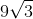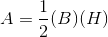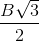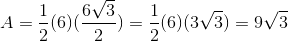# High School Math : How to find the area of a right triangle

## Example Questions

2 Next →

### Example Question #61 : Plane Geometry

The length of one leg of an equilateral triangle is 6. What is the area of the triangle?Explanation:The base is equal to 6.

The height of an quilateral triangle is equal to, whereis the length of the base.2 Next →